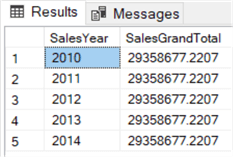# SQL Server Window Functions Examples

##### Overview

Let’s start the tutorial with a couple of T-SQL queries where we use window functions. We’ll use those examples to illustrate the potential power of the functions and how you can use them in your daily programming.

Throughout this tutorial, the AdventureWorks2017 data warehouse is used as a sample database. You can download the database from Github.

If the examples seem a bit complicated, don’t worry, we’ll explain every one of them in this tutorial.

## Example 1 – Calculate Grand Total in SQL Server

Let’s start easy with calculating the grand total of all sales. When using window functions, this grand total will be displayed on each row:

```SELECT DISTINCT
SalesYear          = YEAR([OrderDate])
,SalesGrandTotal    = SUM([SalesAmount]) OVER()
ORDER BY SalesYear;
```

This query returns the following results:The query raises a couple of questions:

• Why did we use DISTINCT?
• Why is the result on each row the same?
• What is OVER()?

The OVER() clause is what makes an ordinary function like SUM a window function. As we’ll see in this tutorial, there are some functions that can only be used as a window function, but other aggregate functions can be used in other scenarios as well. Functions like SUM, AVG, MIN and MAX were being used with the GROUP BY clause years before window functions were introduced.

A window function – SUM in this example – returns a result for each row. Since we calculate the grand total, it’s the same for each row. If we didn’t use the DISTINCT clause, a row would have been returned for every record in the FactInternetSales table.By using DISTINCT, we limit the result set to only the 5 distinct values for the order year.

Calculating the grand total like we did in the query doesn’t seem useful at first, but the result can be used for example to calculate a percentage of total. We’ll look into that use case in the next part of this tutorial.

## Example 2 – Find Top 5 Records in SQL Server

In the next example we’re going to use a typical window function: RANK. This function allows us to find customers who have generated the most sales.

```SELECT
[CustomerAlternateKey]
,[SalesAmount]
,CustomerRank = RANK() OVER (ORDER BY [SalesAmount] DESC)
FROM
( -- first calculate the sales amount for each customer
SELECT
c.[CustomerAlternateKey]
,[SalesAmount] = SUM(f.[SalesAmount])
FROM [dbo].[FactInternetSales]  f
JOIN [dbo].[DimCustomer]        c ON [c].[CustomerKey] = [f].[CustomerKey]
GROUP BY [c].[CustomerAlternateKey]
) tmp;
```

This returns the following result:Since we ordered by descending sales amount in the OVER() clause, the customers with the highest sales amount have are ranked first.

## Example 3 – Calculate the Median Value in SQL Server

Let’s calculate the median salary for the AdventureWorks employee per department. We’ll use the PERCENTILE_CONT function. The 0.5 percentile is the same as the median value.

```SELECT DISTINCT
[DepartmentName]
,MedianRate = PERCENTILE_CONT(0.5) WITHIN GROUP (ORDER BY [BaseRate]) OVER (PARTITION BY [DepartmentName])
FROM [dbo].[DimEmployee]
ORDER BY MedianRate DESC;
```

The result set:The PERCENTILE_CONT function has a more complex syntax than other window functions. We’ll get to the bottom of this in part 8.